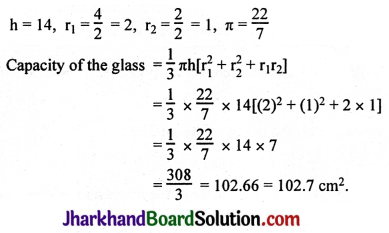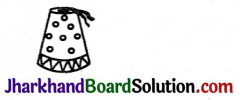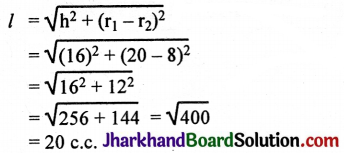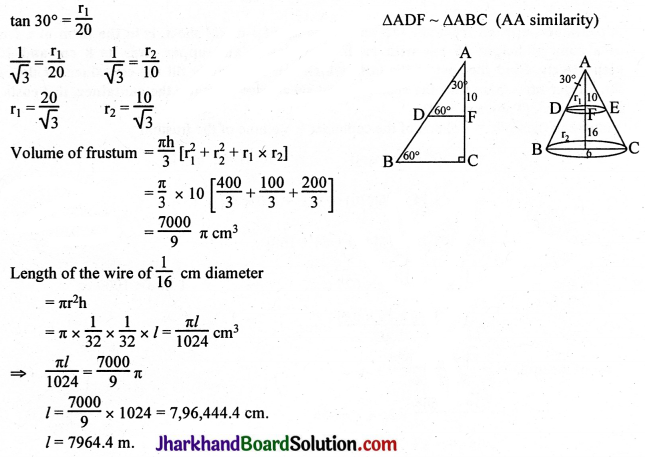# JAC Class 10 Maths Solutions Chapter 13 Surface Areas and Volumes Ex 13.4

Jharkhand Board JAC Class 10 Maths Solutions Chapter 13 Surface Areas and Volumes Ex 13.4 Textbook Exercise Questions and Answers.

## JAC Board Class 10 Maths Solutions Chapter 13 Surface Areas and Volumes Exercise 13.4

Use π = $$\frac{22}{7}$$, unless stated otherwise.

Question 1.
A drinking glass is in the shape of a frustum of a cone of height 14 cm. The diameters of its two circular ends are 4 cm and 2 cm. Find the capacity of the glass.
Solution:Question 2.
The slant height of a frustum of a cone is 4 cm and the perimeters (circumference) of its circular ends are 18 cm and 6 cm. Find the curved surface area of the frustum.
Solution:
C.S.A. of the frustum = πl(r1 + r2)
2πr1 = 18
r1 = $$\frac{18}{2 \pi}=\frac{9}{\pi}$$
2πr2 = 6
r2 = $$\frac{6}{2 \pi}=\frac{3}{\pi}$$
C.S.A. of the frustum = xl(r1 + r2)
= π × $$4\left[\frac{9}{\pi}+\frac{3}{\pi}\right]$$
= π × 4 × $$\frac{12}{\pi}$$
= 48 cm2.3Question 3.
A fez, the cap used by the Turks, is shaped like the frustum of a cone. If its radius on the open side is 10 cm, radius at the upper base is 4 cm and its slant height is 15 cm, find the area of material used for making it.Solution:
r1 = 4, r2 = 10, l = 15
Area of the material used = C.S.A. of the frustum + Area of the circular top
= πl(r1 + r2) + πr12
= $$\frac{22}{7}$$ × 15 (4 + 10) + π × 42
= $$\frac{22}{7}$$ × 15 × 14 + $$\frac{22}{7}$$ × 16
= $$\frac{22}{7}$$(210 + 16)
= $$\frac{22}{7}$$ × 226
= $$\frac{4972}{7}$$
= 710$$\frac{2}{7}$$ cm2.

Question 4.
A container, opened from the top and made up of a metal sheet, is in the form of a frustum of a cone of height 16 cm with radii of its lower and upper ends as 8 cm and 20 cm, respectively. Find the cost of the milk which can completely fill the container, at the rate of Rs. 20 per litre. Also find the cost of metal sheet used to make the container, if it costs Rs. 8 per 100 cm2. (Take π = 3.14).
Solution:
Volume of the milk = Volume of the container = Volume of the frustum
= $$\frac{1}{3}$$πh(r12 + r22 + r1r2)   [r1 = 20, r2 = 8, h = 16]
= $$\frac{1}{3}$$ × 3.14 × 16[(20)2 + (8)2 + (20 × 8)]
= $$\frac{1}{3}$$ × 3.14 × 16[400 + 64 + 160]
= $$\frac{1}{3}$$ × 3.14 × 16 × 624 c.c. [1 litre 1000 cc]
= $$\frac{1}{3}$$ × $$\frac{3.14 \times 16 \times 624}{1000}$$ litres.
Cost of the milk = $$\frac{3.14 \times 16 \times 624}{3 \times 1000}$$
= $$\frac{3.14 \times 1664}{25}$$
= 3.14 × 66.56
= 208.99 = Rs. 209.
Area of the metal sheet used = πl(r1 + r2) + πr22 [r1 = 20, r2 = 8, h = 16]Area of the metal sheet used = πl(r1 + r2) + πr22
= 3.14 × 20(20 + 8) + 3.14 × 82
= 3.14 × 20 × 28 + 3.14 × 64
= 3.14[(20 × 28) + 64]
= 3.14(560 + 64)
= 3.14 × 624 sq. cms.
Cost of the metal sheet = Area × Rate
= 3.14 × 624 × rate
= $$\frac{3.14 \times 624 \times 8}{100}$$
= 3.14 × 624 × 0.08
= 3.14 × 49.92
= 156.7488
= Rs. 156.75.Question 5.
A metallic right circular cone 20 cm high and whose vertical angle is 60° is cut into two parts the middle of its height by a plane parallel to its base. If the frustum so obtained be drawn into a wire of diameter $$\frac{1}{6}$$ cm, find the length of the wire.
Solution: